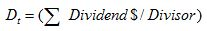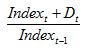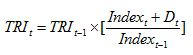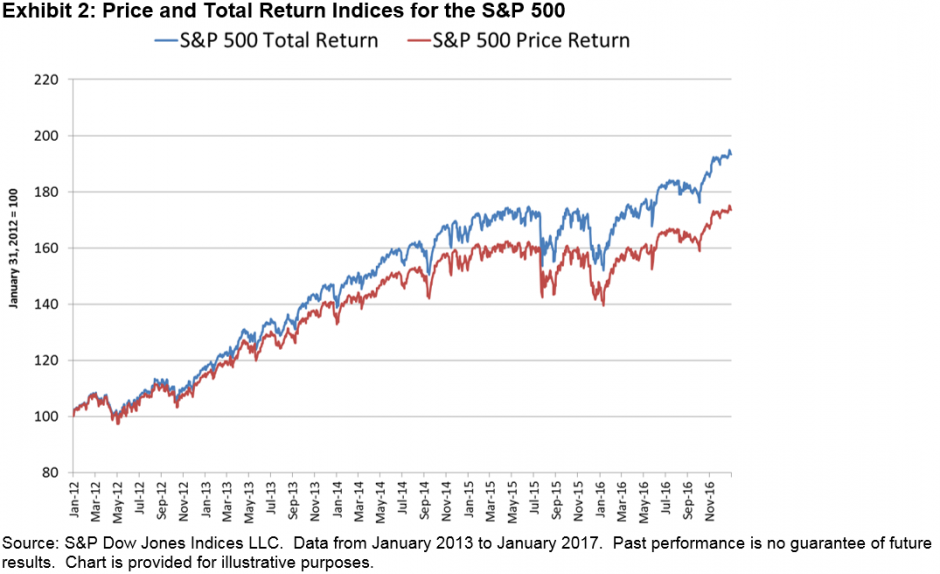# Index Basics: Calculating an Index’s Total Return

Total return indices deserve more attention.  They more closely represent what an investor actually takes home: the return of an index, plus dividends paid and reinvested in the index.  Their better-known counterparts, which only track price changes in securities—often called “price return indices”1—get all the fanfare (see “Dow Hits 20,000 for the First Time”).  Total return indices, on the other hand, are often quietly downloaded and placed in a chart halfway through a financial advisor’s presentation.

Though I doubt people will ever stop looking at price return indices first, a step in the right direction is for investors to develop a better understanding of how total return indices work so they will use them more often.

How Total Return Indices Are Calculated

The aim of a total return index is to reflect the full benefit of holding an index’s constituents over a given time.  This means reinvesting dividends into the index by adding them, period by period, to the price changes of the index portfolio.  But how do you add dividends—which are valued in dollars, euros, and other currencies—to an index, which is expressed in points?

The trick is one you learned in fifth grade, to establish a common denominator.  This is done by dividing the dividends paid over a period by the same divisor used to calculate the index.  This gives you “dividends paid out per index point.”  The equation is as follows.The next step is to adjust the price return index value for the day, not the total return index, using the following formula, which combines the dividends and index price change.Finally, to apply this adjustment to the total return index series, which accounts for a full history of dividend payments, this value is multiplied by the previous day’s total return index level.Again, the process is to (1) find the dividends per index point, (2) adjust the price return index, and then (3) apply this adjustment to the previous day’s total return index value.

The Power of the Total Return

Market participants often underestimate the power of dividends.  Exhibits 1 and 2 show the price and total return indices for the S&P 500® and the Dow Jones Industrial Average®.These charts show five years of index values, ending in January 2017.  By the end of this period, the total return indices for the Dow Jones Industrial Average and S&P 500 were ahead of their price return counterparts by 13.5% and 11.3%, respectively.

The next time an index—likely a price return index—hits a major milestone and is noted in the media, take the time to go to the S&P Dow Jones Indices website to see how the total return version of this same index performed.  With dividends included, the index will have done even better than journalists and the talking heads on television are acknowledging.

1   “Price return indices” should not be confused with “price-weighted indices.”  In price-weighted indices, the most famous of which is the Dow Jones Industrial Average, components are assigned weights according to the level of their individual prices.  A “price return index” is any index with any weighting scheme that only accounts for price changes in the underlying securities.  The DJIA is a price return index and a price-weighted index.  The S&P 500 is a price return index, but market-cap weighted, not price weighted.